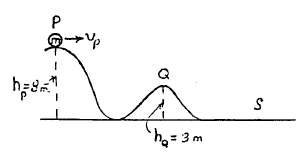# Horizontal velocity over an uneven surface

## Homework Statement

A small ball of m=1kg at height hp = 8m at point p, is given an initial horizontal speed vp = 10 m/s. It slides over an uneven frictionless surface. What is speed vq of the ball at height hq = 3m at point q?## Homework Equations

Ui + KEi = Uf + KEf

## The Attempt at a Solution

I figured out how to find the velocity at point q, but when I first did the problem, I thought that the horizontal velocity wouldn't change because there is no horizontal force acting on the ball. The surface is frictionless, so going back to kinematics, if you throw a ball at an angle, the horizontal velocity doesn't change because gravity only acts downward, so I thought it would remain at 10 m/s. But the speed actually increased to 14 m/s after I solved it using the total energy equation.
Why was my first way wrong?

BvU
•